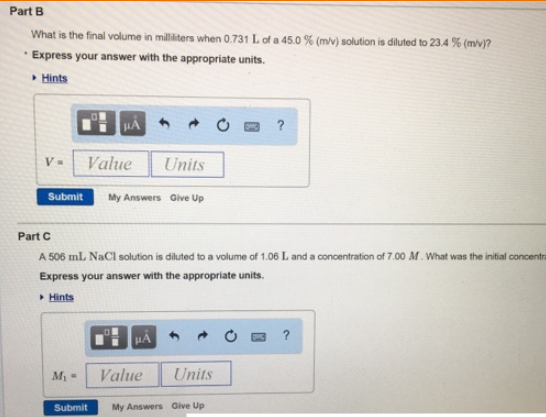# Problem: Part BWhat is the final volume in millilitres when 0.731 L of a 45.0 % (m/v) solution is diluted to 23.4 % (m/v)? Express your answer with the appropriate units.Part C A 506 mL NaCl solution is diluted to a volume of 1.06 L and a concentration of 7.00 M. What mi the initial concentrate? Express your answer with the appropriate units.

###### FREE Expert Solution###### Problem Details

Part B

What is the final volume in millilitres when 0.731 L of a 45.0 % (m/v) solution is diluted to 23.4 % (m/v)?

Part C

A 506 mL NaCl solution is diluted to a volume of 1.06 L and a concentration of 7.00 M. What mi the initial concentrate?